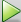## Enable Plotting Using the Simulation Data Inspector

### From the UI

You can use the Simulation Data Inspector to inspect and compare floating-point and fixed-point logged input and output data. In the Fixed-Point Conversion tool:

2. In the Advanced Settings dialog box, set Plot with Simulation Data Inspector to `Yes`.

3. At the Test Numerics stage in the conversion process, click , select ```Log inputs and outputs for comparison plots```, and then click.

For an example, see Propose Fixed-Point Data Types Based on Derived Ranges.

### From the Command Line

You can use the Simulation Data Inspector to inspect and compare floating-point and fixed-point input and output data logged using the function. At the MATLAB® command line:

1. Create a fixed-point configuration object and configure the test file name.

```fixptcfg = coder.config('fixpt'); fixptcfg.TestBenchName = 'dti_test'; ```

2. Select to run the test file to verify the generated fixed-point MATLAB code. Log inputs and outputs for comparison plotting and select to use the Simulation Data Inspector to plot the results.

```fixptcfg.TestNumerics = true; fixptcfg.LogIOForComparisonPlotting = true; fixptcfg.PlotWithSimulationDataInspector = true;```
3. Generate fixed-point MATLAB code using `codegen`.

`codegen -float2fixed fixptcfg -config cfg dti`

For an example, see Propose Fixed-Point Data Types Based on Derived Ranges.